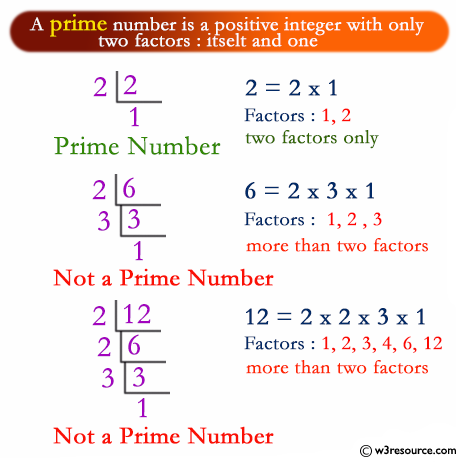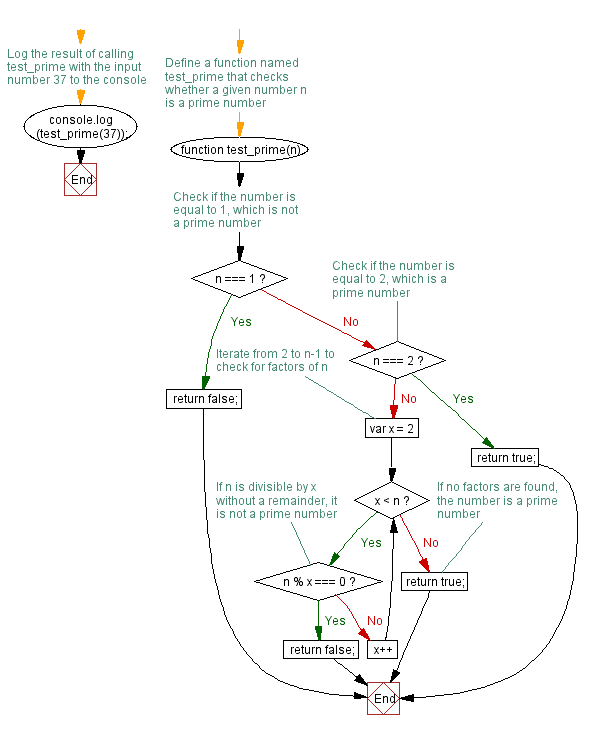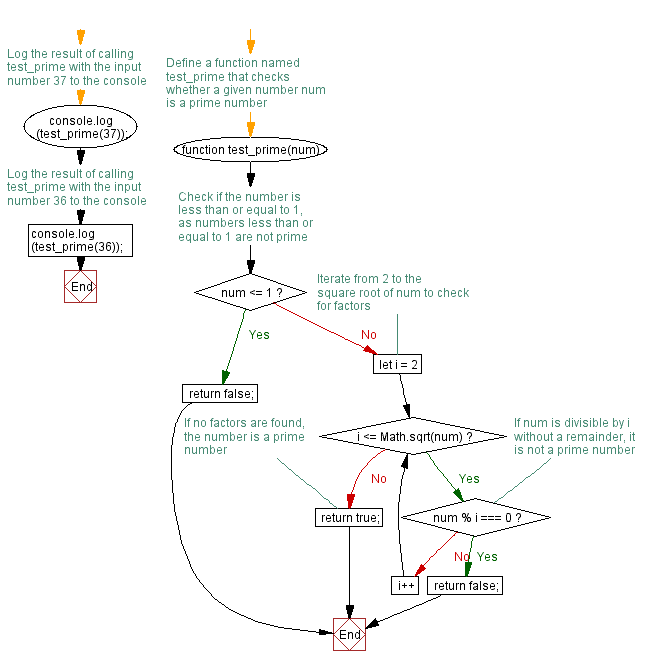# JavaScript: Check a number is prime or not

## JavaScript Function: Exercise-8 with Solution

Write a JavaScript function that accepts a number as a parameter and checks whether it is prime or not.

Note : A prime number (or a prime) is a natural number greater than 1 that has no positive divisors other than 1 and itself.

Pictorial Presentation:Sample Solution-1:

JavaScript Code:

``````function test_prime(n)
{

if (n===1)
{
return false;
}
else if(n === 2)
{
return true;
}else
{
for(var x = 2; x < n; x++)
{
if(n % x === 0)
{
return false;
}
}
return true;
}
}

console.log(test_prime(37));
```
```

Sample Output:

```true
```

Flowchart:Live Demo:

See the Pen javascript-function-exercise-8 by w3resource (@w3resource) on CodePen.

Improve this sample solution and post your code through Disqus

Sample Solution-2:

JavaScript Code:

``````function test_prime(num) {
if (num <= 1) {
return false;
}
for (let i = 2; i <= Math.sqrt(num); i++) {
if (num % i === 0) {
return false;
}
}
return true;
}
console.log(test_prime(37));
console.log(test_prime(36));
```
```

Sample Output:

```true
false
```

Explanation:
The above function first checks if the input number is less than or equal to 1, which is not a prime number, and immediately returns false if it is. It then loops through all numbers from 2 to the square root of the input number using a for loop. It uses the modulus operator to check if each number is divisible by that number. If the input number is divisible by any number other than 1 and itself, it is not a prime number and the function returns false. Upon completion of the loop without finding a divisor, the function returns true, indicating that the input number is prime.

Flowchart:Live Demo:

See the Pen javascript-function-exercise-8-1 by w3resource (@w3resource) on CodePen.

Improve this sample solution and post your code through Disqus

What is the difficulty level of this exercise?

Test your Programming skills with w3resource's quiz.

﻿